# NCERT Solutions class 7 Maths Chapter-11 Exercise 11.2

## NCERT Solutions class 7 Maths Chapter-11 Perimeter and Area

### Perimeter and Area Exercise-11.2

NCERT Solutions Class-7 Maths chapter-11 Perimeter and Area Exercise-11.2 is prepared by academic team of pw all the questions of NCERT text book are solved step by step with proper and detail solutions explaining each and every questions . For More and additional questions of CBSE class 7 maths you can go to class 7 maths sections. NCERT class 7 Maths Solutions is the best way to enhanced your mathematics skill. And pw practice worksheet & question bank will help you a lot .

### Solutions of Chapter Perimeter and Area Exercise-11.2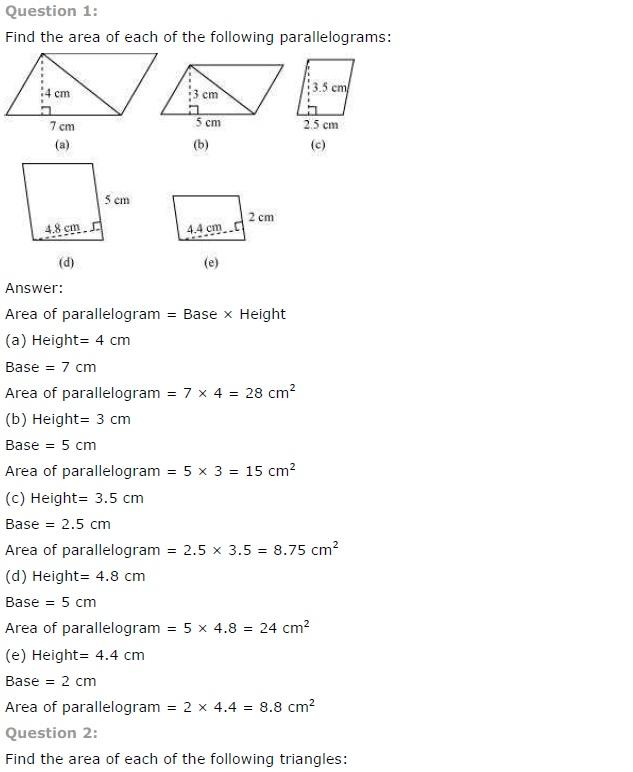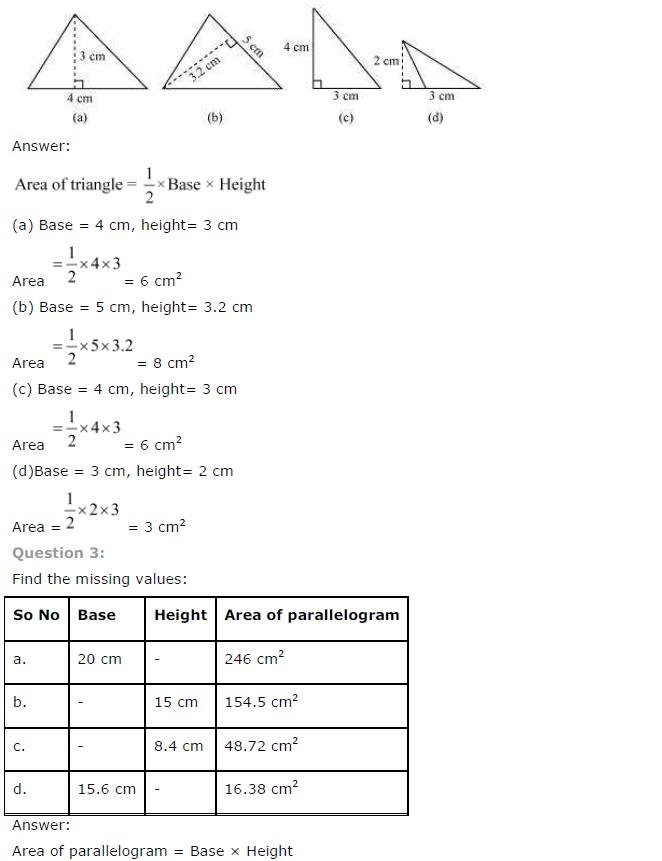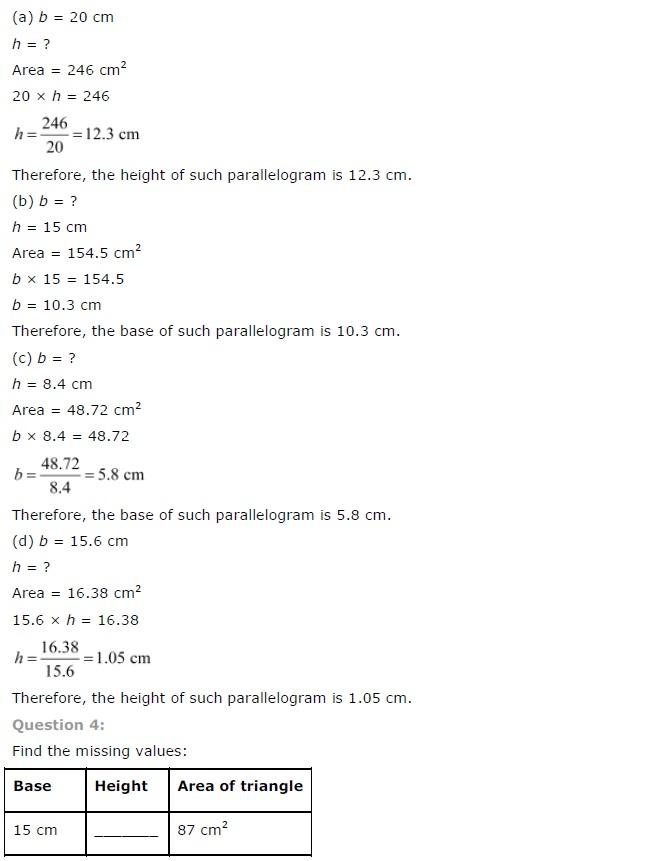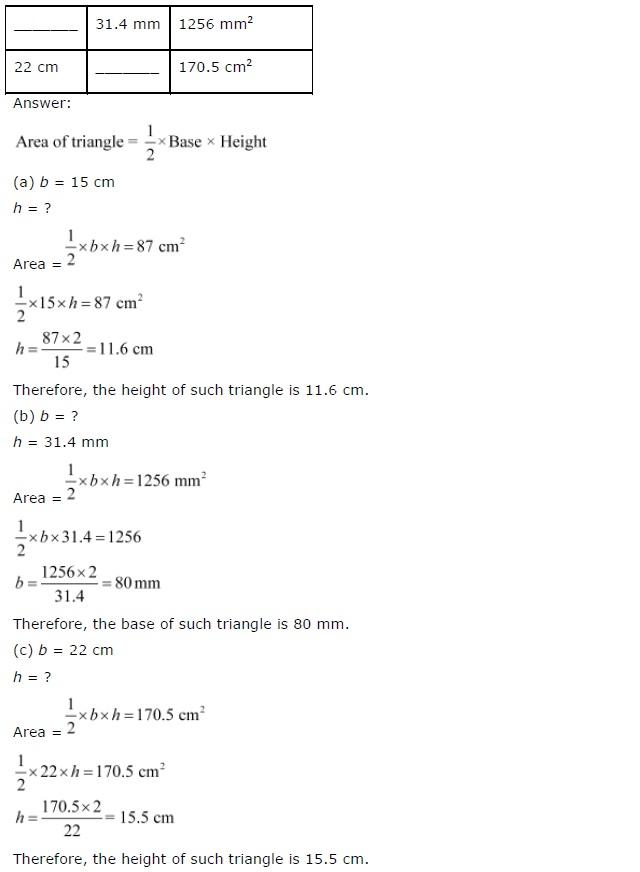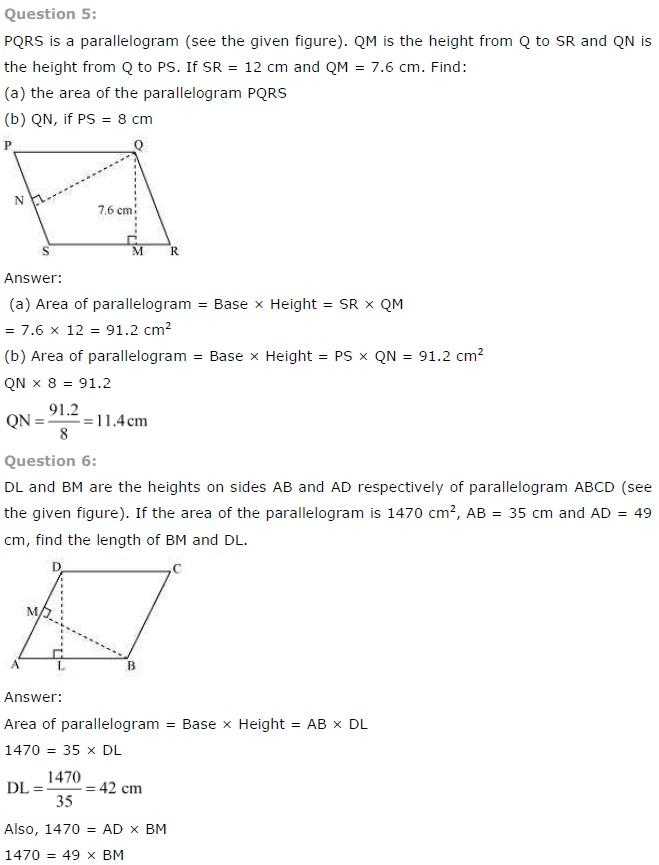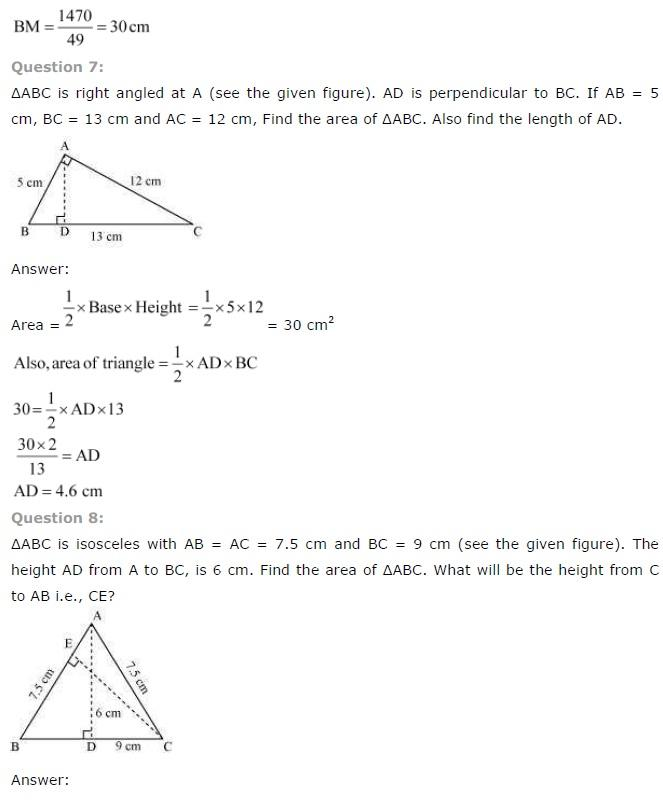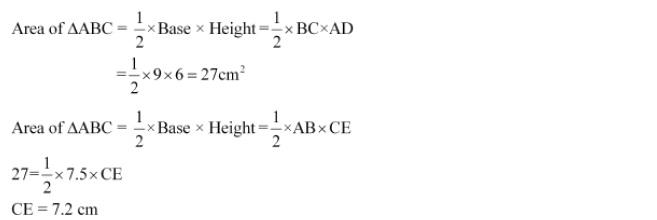NCERT CLASS 7 MATHEMATICS SOLUTIONS

NCERT Solutions for Class 7 Maths Chapter 1 - Integers

NCERT Solutions for Class 7 Maths Chapter 2 - Fractions and Decimals

NCERT Solutions for Class 7 Maths Chapter 3 - Data Handling

NCERT Solutions for Class 7 Maths Chapter 4 - Simple Equations

NCERT Solutions for Class 7 Maths Chapter 5 - Lines and Angles

NCERT Solutions for Class 7 Maths Chapter 6 - The Triangle and Its Properties

NCERT Solutions for Class 7 Maths Chapter 7 - Congruence of Triangles

NCERT Solutions for Class 7 Maths Chapter 8 - Comparing Quantities

NCERT Solutions for Class 7 Maths Chapter 9 - Rational Numbers

NCERT Solutions for Class 7 Maths Chapter 10 - Practical Geometry

NCERT Solutions for Class 7 Maths Chapter 11 - Perimeter and Area

NCERT Solutions for Class 7 Maths Chapter 12 - Algebraic Expressions

NCERT Solutions for Class 7 Maths Chapter 13 - Exponents and Powers

NCERT Solutions for Class 7 Maths Chapter 14 - Symmetry

NCERT Solutions for Class 7 Maths Chapter 15 - Visualising Solid Shapes